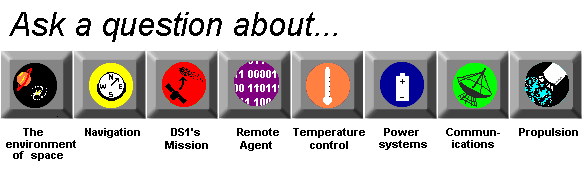Communications System

How are frequency and wavelength related?

Electromagnetic waves always travel at the same speed (299,792 km per second). This is one of their defining characteristics. In the electromagnetic spectrum there are many different types of waves with varying frequencies and wavelengths. They are all related by one important equation: Any electromagnetic wave's frequency multiplied by its wavelength equals the speed of light.

FREQUENCY OF OSCILLATION x WAVELENGTH = SPEED OF LIGHT

We can use this relationship to figure out the wavelength or frequency of any electromagnetic wave if we have the other measurement. Just divide the speed of light by whichever measurement you have and then you've got the other.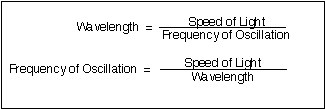Figure from NASA's Jet Propulsion Laboratory's publication: Basics of Space Flight Learner's Workbook. http://www-b.jpl.nasa.gov/basics/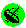What are radio waves?What is frequency?How is data put on radio waves?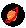Is there energy in space?How does reflection affect radio waves?More about radio waves.What is electromagnetic radiation?What radio frequency does DS1 use for communications?How do you make a radio wave?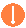What makes EM radiation?